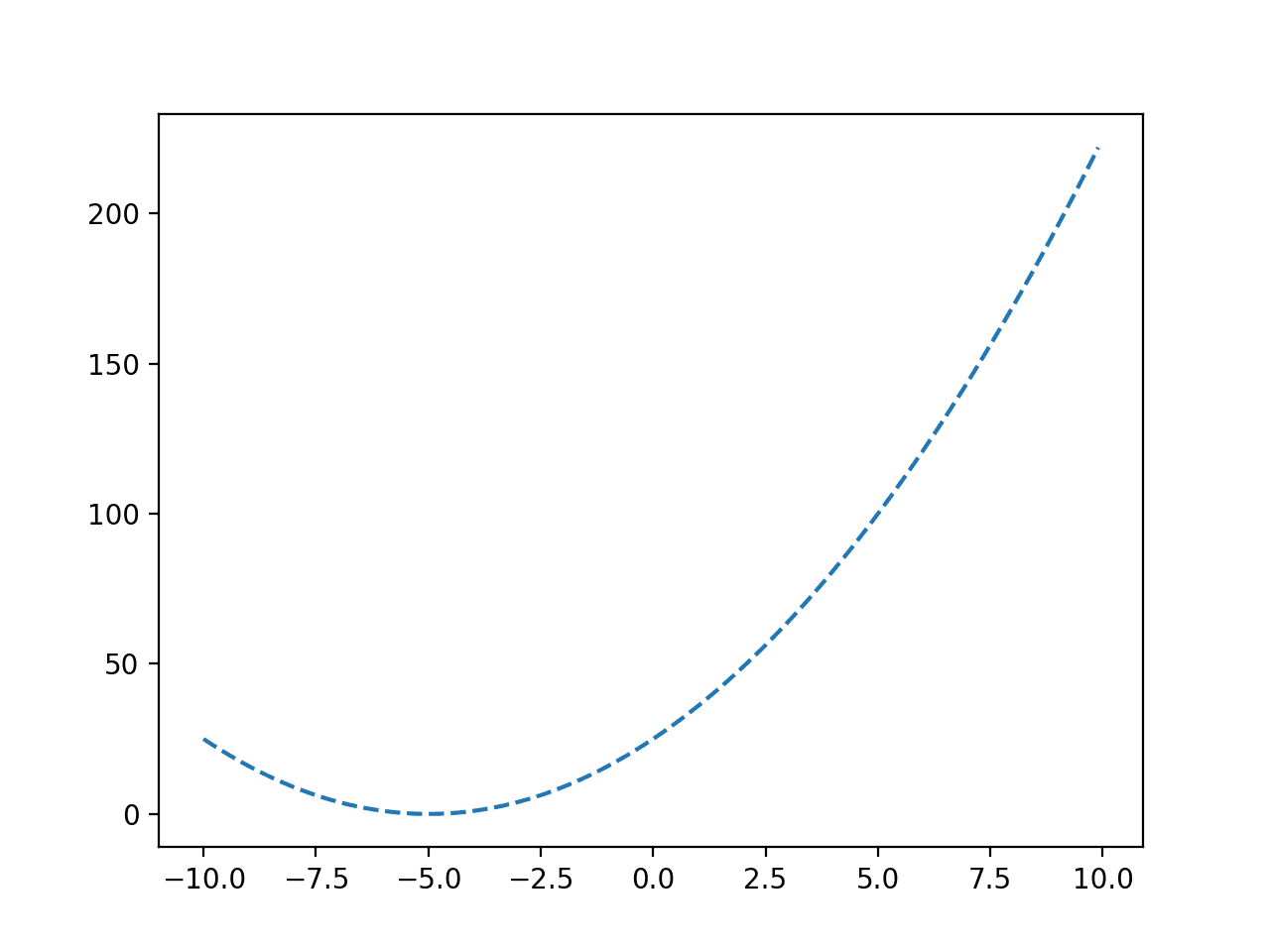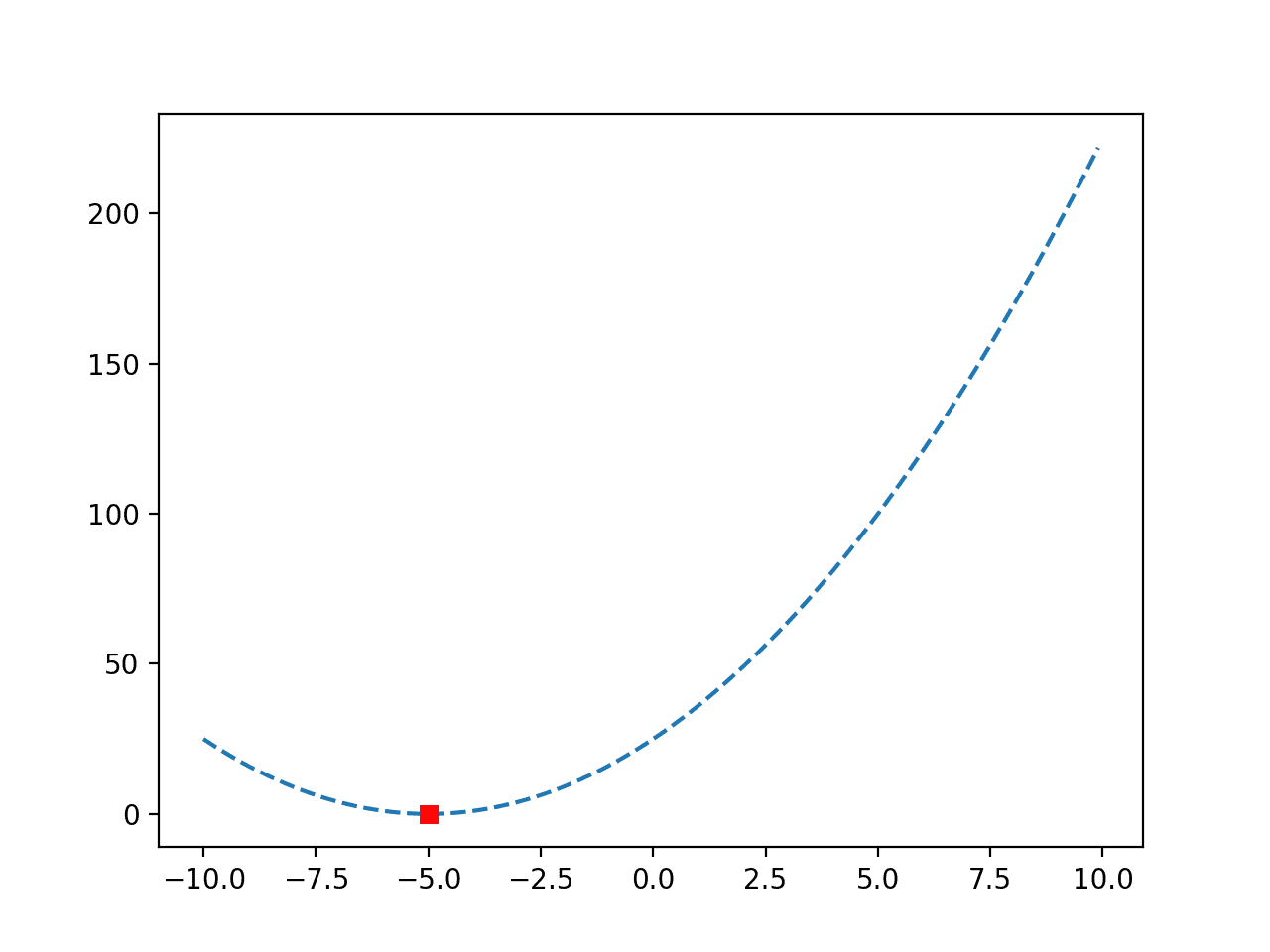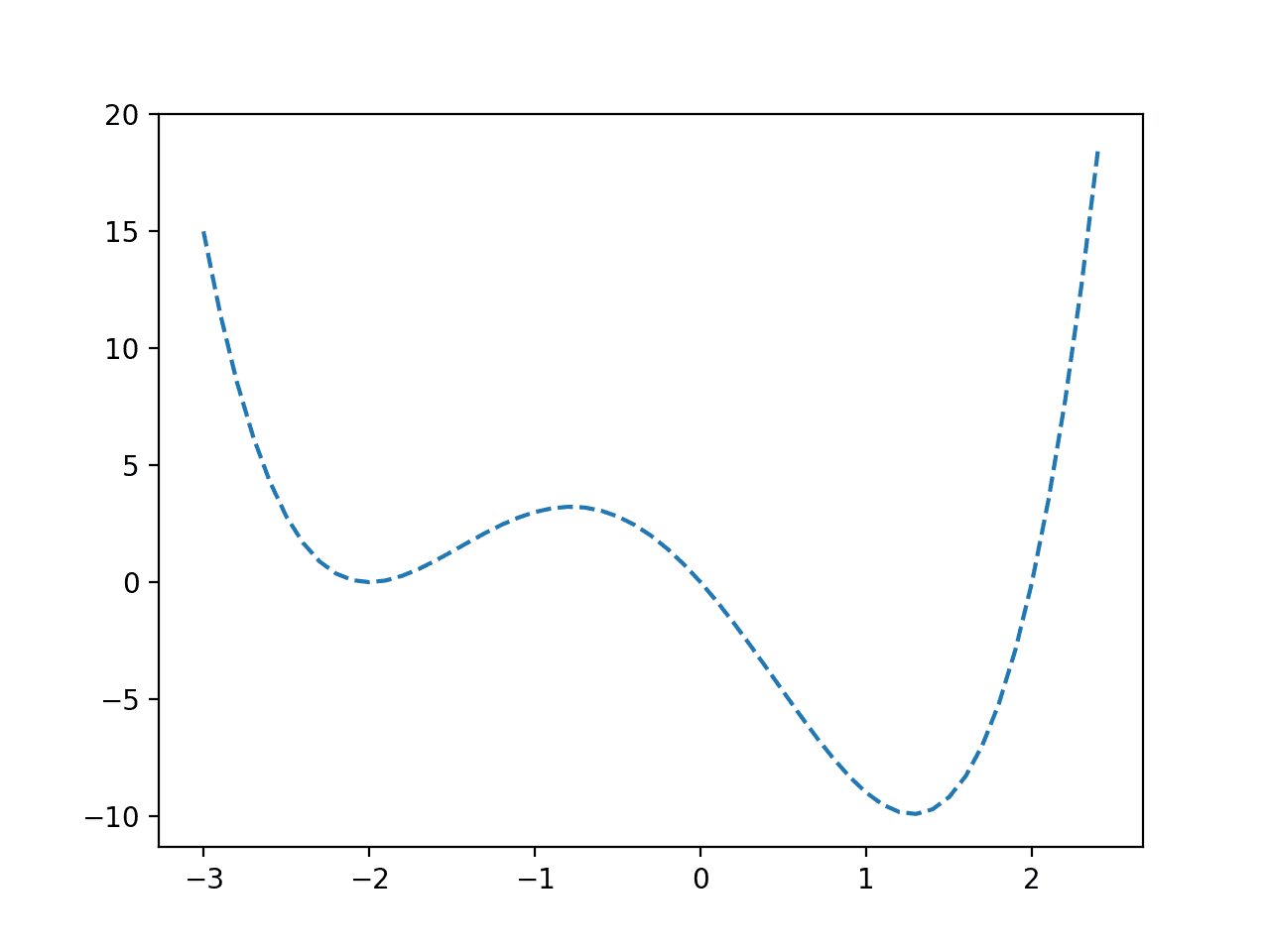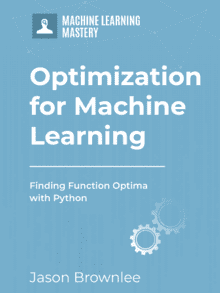# Univariate Function Optimization in Python

Last Updated on October 12, 2021

#### How to Optimize a Function with One Variable?

Univariate function optimization involves finding the input to a function that results in the optimal output from an objective function.

This is a common procedure in machine learning when fitting a model with one parameter or tuning a model that has a single hyperparameter.

An efficient algorithm is required to solve optimization problems of this type that will find the best solution with the minimum number of evaluations of the objective function, given that each evaluation of the objective function could be computationally expensive, such as fitting and evaluating a model on a dataset.

This excludes expensive grid search and random search algorithms and in favor of efficient algorithms like Brent’s method.

In this tutorial, you will discover how to perform univariate function optimization in Python.

After completing this tutorial, you will know:

• Univariate function optimization involves finding an optimal input for an objective function that takes a single continuous argument.
• How to perform univariate function optimization for an unconstrained convex function.
• How to perform univariate function optimization for an unconstrained non-convex function.

Kick-start your project with my new book Optimization for Machine Learning, including step-by-step tutorials and the Python source code files for all examples.

Let’s get started.Univariate Function Optimization in Python
Photo by Robert Haandrikman, some rights reserved.

## Tutorial Overview

This tutorial is divided into three parts; they are:

1. Univariate Function Optimization
2. Convex Univariate Function Optimization
3. Non-Convex Univariate Function Optimization

## Univariate Function Optimization

We may need to find an optimal value of a function that takes a single parameter.

In machine learning, this may occur in many situations, such as:

• Finding the coefficient of a model to fit to a training dataset.
• Finding the value of a single hyperparameter that results in the best model performance.

This is called univariate function optimization.

We may be interested in the minimum outcome or maximum outcome of the function, although this can be simplified to minimization as a maximizing function can be made minimizing by adding a negative sign to all outcomes of the function.

There may or may not be limits on the inputs to the function, so-called unconstrained or constrained optimization, and we assume that small changes in input correspond to small changes in the output of the function, e.g. that it is smooth.

The function may or may not have a single optima, although we prefer that it does have a single optima and that shape of the function looks like a large basin. If this is the case, we know we can sample the function at one point and find the path down to the minima of the function. Technically, this is referred to as a convex function for minimization (concave for maximization), and functions that don’t have this basin shape are referred to as non-convex.

• Convex Target Function: There is a single optima and the shape of the target function leads to this optima.

Nevertheless, the target function is sufficiently complex that we don’t know the derivative, meaning we cannot just use calculus to analytically compute the minimum or maximum of the function where the gradient is zero. This is referred to as a function that is non-differentiable.

Although we might be able to sample the function with candidate values, we don’t know the input that will result in the best outcome. This may be because of the many reasons it is expensive to evaluate candidate solutions.

Therefore, we require an algorithm that efficiently samples input values to the function.

One approach to solving univariate function optimization problems is to use Brent’s method.

Brent’s method is an optimization algorithm that combines a bisecting algorithm (Dekker’s method) and inverse quadratic interpolation. It can be used for constrained and unconstrained univariate function optimization.

The Brent-Dekker method is an extension of the bisection method. It is a root-finding algorithm that combines elements of the secant method and inverse quadratic interpolation. It has reliable and fast convergence properties, and it is the univariate optimization algorithm of choice in many popular numerical optimization packages.

— Pages 49-51, Algorithms for Optimization, 2019.

Bisecting algorithms use a bracket (lower and upper) of input values and split up the input domain, bisecting it in order to locate where in the domain the optima is located, much like a binary search. Dekker’s method is one way this is achieved efficiently for a continuous domain.

Dekker’s method gets stuck on non-convex problems. Brent’s method modifies Dekker’s method to avoid getting stuck and also approximates the second derivative of the objective function (called the Secant Method) in an effort to accelerate the search.

As such, Brent’s method for univariate function optimization is generally preferred over most other univariate function optimization algorithms given its efficiency.

Brent’s method is available in Python via the minimize_scalar() SciPy function that takes the name of the function to be minimized. If your target function is constrained to a range, it can be specified via the “bounds” argument.

It returns an OptimizeResult object that is a dictionary containing the solution. Importantly, the ‘x‘ key summarizes the input for the optima, the ‘fun‘ key summarizes the function output for the optima, and the ‘nfev‘ summarizes the number of evaluations of the target function that were performed.

Now that we know how to perform univariate function optimization in Python, let’s look at some examples.

### Want to Get Started With Optimization Algorithms?

Take my free 7-day email crash course now (with sample code).

Click to sign-up and also get a free PDF Ebook version of the course.

## Convex Univariate Function Optimization

In this section, we will explore how to solve a convex univariate function optimization problem.

First, we can define a function that implements our function.

In this case, we will use a simple offset version of the x^2 function e.g. a simple parabola (u-shape) function. It is a minimization objective function with an optima at -5.0.

We can plot a coarse grid of this function with input values from -10 to 10 to get an idea of the shape of the target function.

The complete example is listed below.

Running the example evaluates input values in our specified range using our target function and creates a plot of the function inputs to function outputs.

We can see the U-shape of the function and that the objective is at -5.0.Line Plot of a Convex Objective Function

Note: in a real optimization problem, we would not be able to perform so many evaluations of the objective function so easily. This simple function is used for demonstration purposes so we can learn how to use the optimization algorithm.

Next, we can use the optimization algorithm to find the optima.

Once optimized, we can summarize the result, including the input and evaluation of the optima and the number of function evaluations required to locate the optima.

Finally, we can plot the function again and mark the optima to confirm it was located in the place we expected for this function.

The complete example of optimizing an unconstrained convex univariate function is listed below.

Running the example first solves the optimization problem and reports the result.

Note: Your results may vary given the stochastic nature of the algorithm or evaluation procedure, or differences in numerical precision. Consider running the example a few times and compare the average outcome.

In this case, we can see that the optima was located after 10 evaluations of the objective function with an input of -5.0, achieving an objective function value of 0.0.

A plot of the function is created again and this time, the optima is marked as a red square.Line Plot of a Convex Objective Function with Optima Marked

## Non-Convex Univariate Function Optimization

A convex function is one that does not resemble a basin, meaning that it may have more than one hill or valley.

This can make it more challenging to locate the global optima as the multiple hills and valleys can cause the search to get stuck and report a false or local optima instead.

We can define a non-convex univariate function as follows.

We can sample this function and create a line plot of input values to objective values.

The complete example is listed below.

Running the example evaluates input values in our specified range using our target function and creates a plot of the function inputs to function outputs.

We can see a function with one false optima around -2.0 and a global optima around 1.2.Line Plot of a Non-Convex Objective Function

Note: in a real optimization problem, we would not be able to perform so many evaluations of the objective function so easily. This simple function is used for demonstration purposes so we can learn how to use the optimization algorithm.

Next, we can use the optimization algorithm to find the optima.

As before, we can call the minimize_scalar() function to optimize the function, then summarize the result and plot the optima on a line plot.

The complete example of optimization of an unconstrained non-convex univariate function is listed below.

Running the example first solves the optimization problem and reports the result.

### Want to Get Started With Ensemble Learning?

Take my free 7-day email crash course now (with sample code).

Click to sign-up and also get a free PDF Ebook version of the course.

In this case, we can see that the optima was located after 15 evaluations of the objective function with an input of about 1.28, achieving an objective function value of about -9.91.

A plot of the function is created again, and this time, the optima is marked as a red square.

We can see that the optimization was not deceived by the false optima and successfully located the global optima.Line Plot of a Non-Convex Objective Function with Optima Marked

This section provides more resources on the topic if you are looking to go deeper.

## Summary

In this tutorial, you discovered how to perform univariate function optimization in Python.

Specifically, you learned:

• Univariate function optimization involves finding an optimal input for an objective function that takes a single continuous argument.
• How to perform univariate function optimization for an unconstrained convex function.
• How to perform univariate function optimization for an unconstrained non-convex function.

Do you have any questions?

## Get a Handle on Modern Optimization Algorithms!#### Develop Your Understanding of Optimization

...with just a few lines of python code

Discover how in my new Ebook:
Optimization for Machine Learning

It provides self-study tutorials with full working code on:
Gradient Descent, Genetic Algorithms, Hill Climbing, Curve Fitting, RMSProp, Adam, and much more...

### 6 Responses to Univariate Function Optimization in Python

1.Saket January 8, 2021 at 5:57 am #

Why don’t you write a formal book on optimization for ml ? I have your all book on ml but optimization part Is missing .

•Jason Brownlee January 8, 2021 at 7:42 am #

Great suggestion. I have one in the works.

2.Sang January 9, 2021 at 3:16 am #

“A convex function is one that does not resemble a basin”. did you meant “A non-convex function is one that does not resemble a basin”.

yes, a book on optimization from your side will be great! and thanks for your works..

3.Vinicius Wittig January 9, 2021 at 10:32 pm #

I agree with the idea of an optimization book! 🙂

•Jason Brownlee January 10, 2021 at 5:42 am #

Thanks.